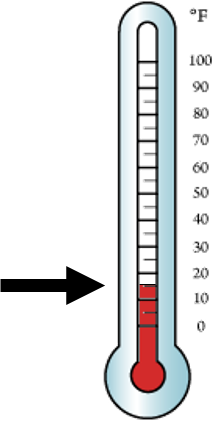1
visibility

What is the temperature shown on the given thermometer?• \$latex 5^\circ C\$

• \$latex 10^\circ F\$

• \$latex 15^\circ F\$

The correct answer is  15°F.

Solution:

First, we find the point where the thermometer changes from red to white.Then, we look at the number to the right.

The temperature is  15°F  as desired.